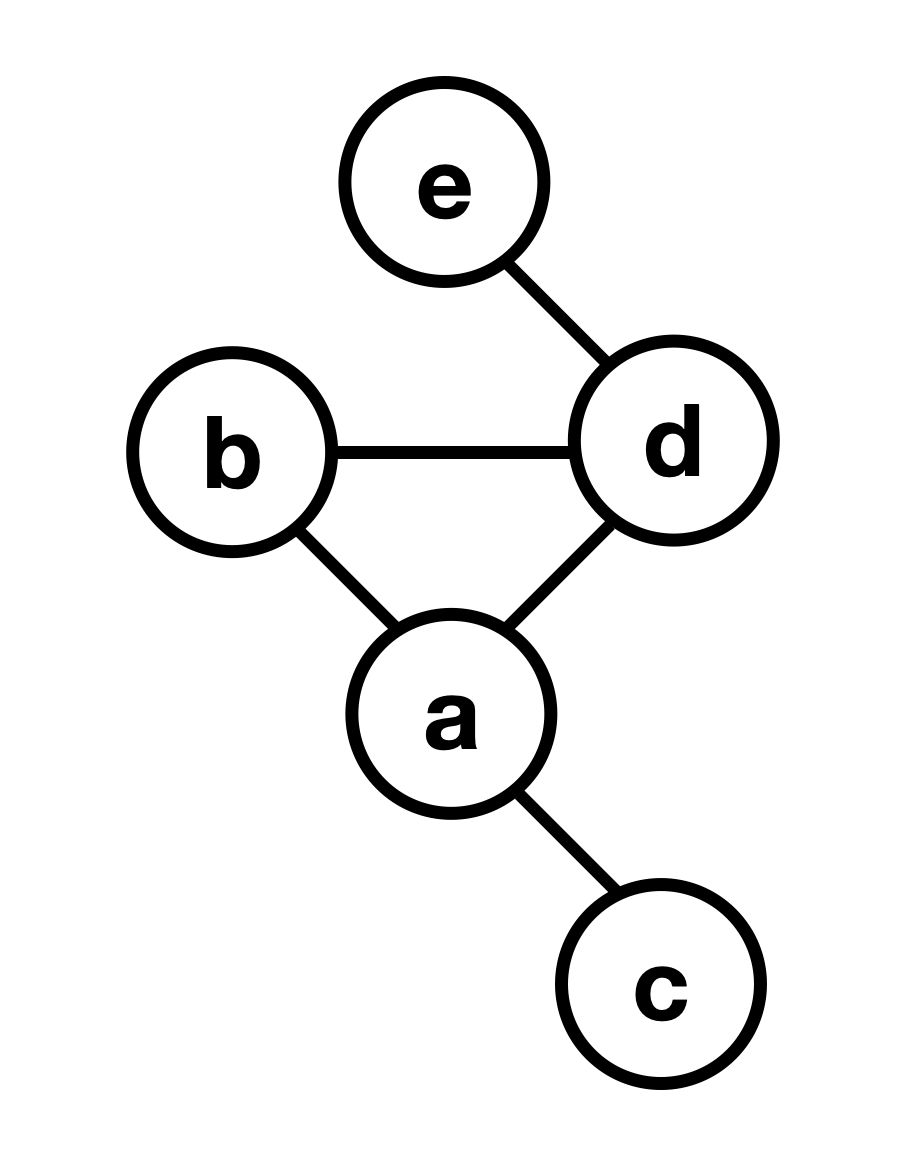Implement a Graph Data Structure | Skilled.dev
Interview Question

# Implement a Graph

We will implement a graph using an . We will assume the `data` contained in vertexes (nodes) is unique, and this value will represent the key in the adjacency list as well.

`` ``When we initialize our graph, we will allow the user to pass a boolean value to indicate if it is undirected. This value will default to true.

We will implement methods to create vertexes, add edges to connect them, and remove a vertex. The implementation will take the following shape:

`` ``

### Note: Graph as Nodes

`` ``

Another common way to work with graphs is directly through nodes. You'll gain a deep understanding of how to do this as you work through this module, so we are looking at another common implementation in this lesson.

## Add a Vertex

This method will add a node to the adjacency matrix. Initially, this node is not connected to any other items.

`` ``

## Add an Edge

Adding an edge is how we indicate nodes are connected. If our graph is undirected, we also need to add a reciprocal edge pointing back.

`` ``

## Remove a Vertex

To delete a vertex, we remove it from the adjacency list. We also must delete all the edges that point to it.

`` ``

## Solution

With that, we have a complete graph implementation that is clean and simple. You can explore it further and run code in the REPL.

`` ``
Ready for the next step?
Find a Job